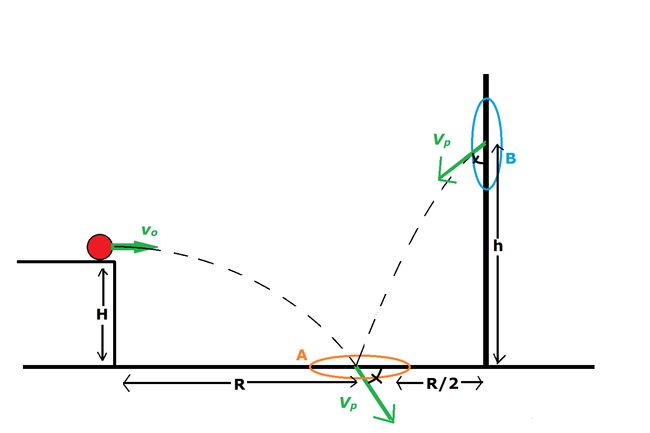# Kinematics With PortalsIf you have never played the video game Portal, a portal is essentially a wormhole (or tunnel) which can teleport an object from one location to another instantaneously, almost as if the object had simply passed through a door. The portal will conserve kinetic energy and speed. However, the object's direction of travel will get shifted depending on how the portals are oriented relative to each other. In math-speak, the object's velocity vector relative to the first portal is equal to that same object's velocity vector relative to the second portal.

Now for the problem:

A ball is launched horizontally off of a ledge of height $H$ at an initial speed of $V_o$. Portal A (orange) is placed on the ground at a distance $R$ such that the ball will pass directly through the portal's center.

On a wall a distance $\dfrac{R}{2}$ away, Portal B (blue) is placed at a height $h$ such that, after the ball passes through, it will again fall into Portal A, but from the other side.

Note that the angle at which the ball falls into Portal A (relative to the ground) is the same as the angle at which the ball falls out of Portal B (relative to the wall).

Given that $H = 6.00 \text{ m}$ and $V_o = 2.53\text{ m/s}$, find $h$.

Give your answer in meters, rounded to 3 significant figures.

Take the acceleration due to gravity as $g = 9.81 \text{ m/s}^2$.

×

Problem Loading...

Note Loading...

Set Loading...